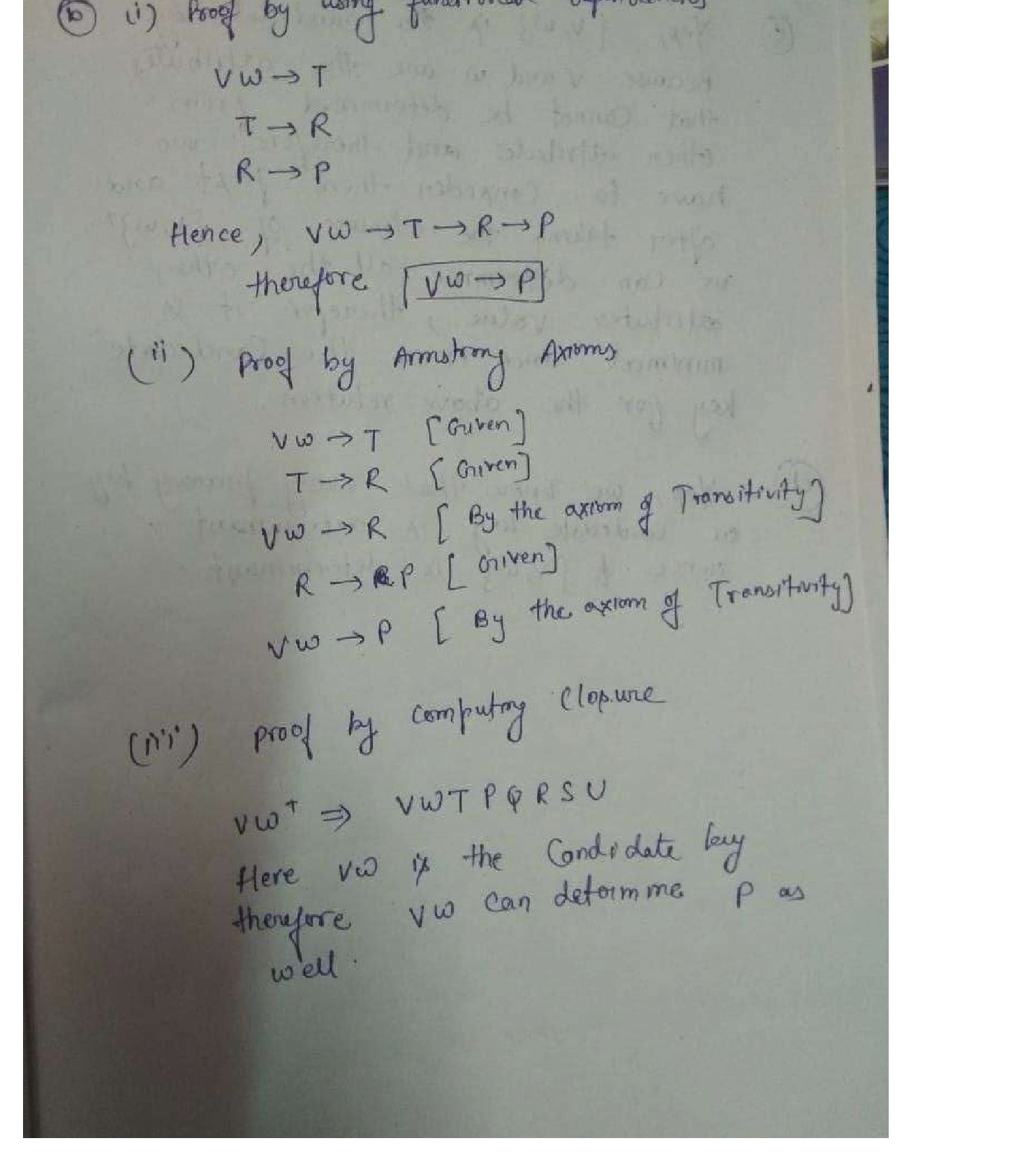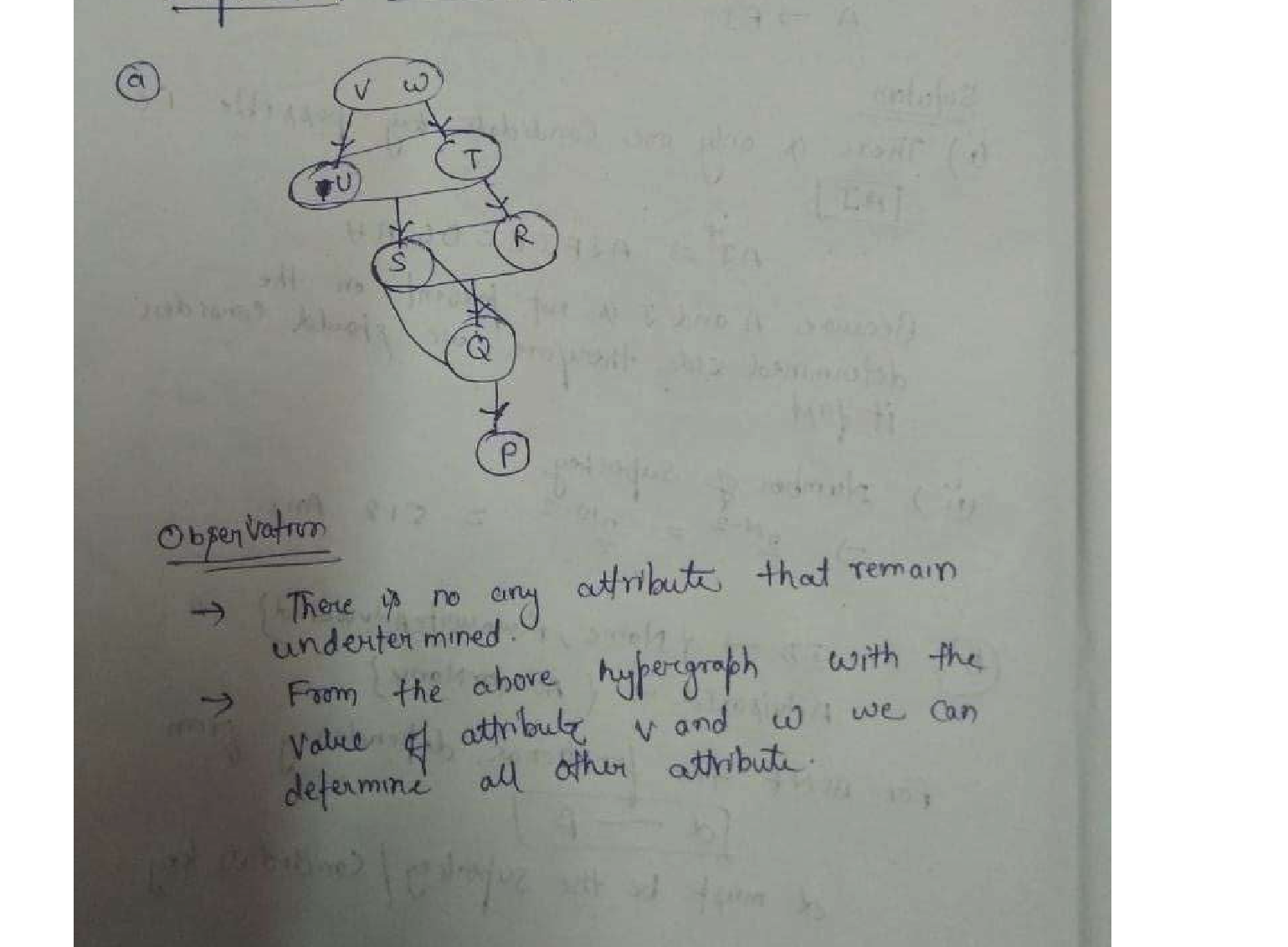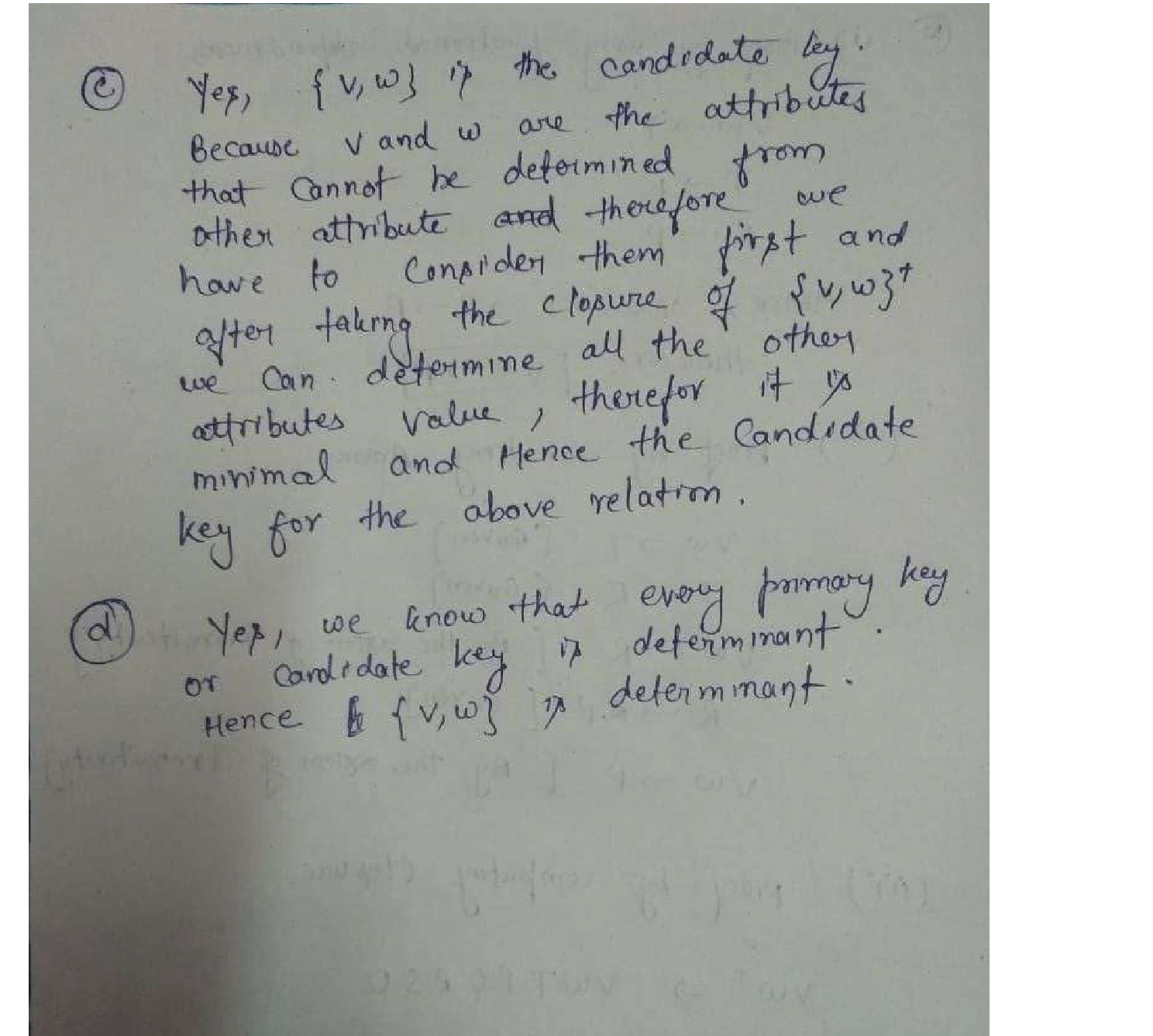Question

Software Engineering

Consider the following set of functional dependencies FA which hold for relation A containing attributes A = {P,Q, R, S, T,U, V, W}:

(a) Visualize FA as a hypergraph as shown in the lecture slides [p. 608]. Make sure that the layout is not too messy and that it is clear which subsets are connected by each arrow. What do you notice?

(b) Prove FAE VW → P, i.e., "every database instance that satisfies FA also satisfies VW → P" (the rhs is an implied FD), by each of the following three methods:

i. Proof by using the definition of functional dependencies.

ii. Proof by using the Armstrong Axioms.

iii. Proof by computing the (attribute) cover {V, W }+ (p. 612f).

(c) Is {V, W } a key of A? Justify your answer briefly.

(d) Is {V, W } a determinant for {P,Q, R, S,T,U, V}? Explain your answer.Verified### Question 45626Software Engineering

Plot time series for all of the variables (variable 1, 2, 3)
10. Time Series Analysis
Check and evaluate auto correlation and partial autocorrelation function for all of the variables (variable 1, 2, 3). Explain the results.
Draw five-point moving average for variable 1 and show the moving average with red colour.
Check seasonality with cycles (sin, cos) and evaluate regression result.
Check residuals to look for patterns after you run the regression for seasonality. Explain the results.

### Question 45625Software Engineering

Draw box-plot of all variables (variable 1, 2, 3) in a single graph.
Check and evaluate ANOVA for all of the variables (variable 1, 2, 3) at the %95 confidence interval. Note that you need to prepare txt file that contains type as 1, 2, 3.Such as
1 2 3
Varl Var2 Var3
Explain the results.

### Question 45624Software Engineering

Compare and evaluate equality of variance for Variable 2 and 3 f-test, Bartlett test, and Fligner test at the %95 confidence interval. Explain the results.
Compare and evaluate equality of Variable 2 and 3 with student-t test at the %95 confidence interval. Explain the results.
Compare and evaluate equality of Variable 2 and 3 with Wilcoxon rank-sum test at the %95 confidence interval. Explain the results.

### Question 45623Software Engineering

Provide the results for y= a + blx1+b2x2+e for your data.
xl is the independent variable in simple linear regression.
x2 is the second independent variable.
Explain the results.

### Question 45622Software Engineering

Provide the results for y = a + blx1+b2x1^2+e for your data. y=dependent variable, x1=independent variable 1, x1^2=square form of independent variable 1. Explain the results.

### Question 45621Software Engineering

Provide the results for y = a + bx1+e for your data. y=dependent variable, xl=independent variable 1. Explain the results.

### Question 45620Software Engineering

Draw qqline for the dependent and the independent variables.
Show skewness and Kustosis values for the dependent and the independent variables.
Apply Shapiro-Wilk normality test. Are the dependent and the independent variables normally distributed? Explain.

### Question 45619Software Engineering

scatterplot of your dependent variable against independent variable 1. Explain the graph.

### Question 45618Software Engineering

Graph your dependent and independent variables using line graph with red colour.
Graph your dependent and independent variables using bar graph with red colour.

### Question 45617Software Engineering

Provide descriptive statistics for the dependent variable.

### Submit query